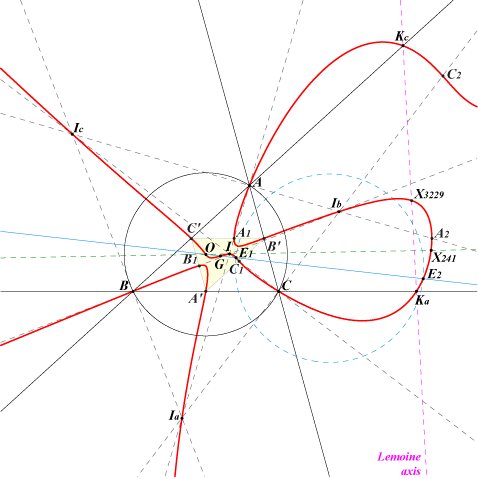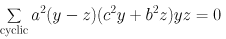X(1), X(2), X(3), X(241), X(3229), X(5000), X(5001) A', B', C' : midpoints of ABC Ia, Ib, Ic : excenters Ka, Kb, Kc : centers of Apollonian circles E1, E2 on the figure are X(5000), X(5001)The tangents at A, B, C are the medians and those at the in/excenters pass through O. The fourth point on AI is A1 = b + c : b : c and the fourth point on IbIc is A2 = b - c : b : -c. These two points lie on the sideline B'C' of the medial triangle and the points B1, B2, C1, C2 are defined similarly. Locus properties : Q026 is the locus of point P such that the anticevian and pedal triangles of P are orthologic. the anticevian and reflection triangles of P are orthologic. the circumcevian triangle of P and the anticevian triangle of the isogonal conjugate of P are perspective. the circumcevian triangle of P and the circumanticevian triangle of the isogonal conjugate of P are perspective. the circumcevian triangle of P and the medial triangle are perspective, together with the circumcircle (Angel Montesdeoca, 2022-10-26). The locus of the perspector is a complicated 10th degree curve. Let PaPbPc be the circumcevian triangle of P and let Ka, Kb, Kc be the centroids of PaBC, PbCA, PcAB respectively. PaPbPc and KaKbKc are perspective if and only if P lies on Q026. Q026 is the quartic Q(X2) mentioned in page K1065 where other examples are given. Q026 is a member of a family of quartics detailed at the bottom of page Q023.